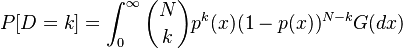# Difference between revisions of "Vasicek Distribution"

## Definition

The Vasicek Distribution is a special probability distribution that emerges in the context of Threshold Models used in credit portfolio modelling. It was first introduced in. There are two versions, the finite portfolio case and its limiting case wher the number of exposures in a portfolio assumed infinitely many / infinitely small.

## Finite Portfolio Case

In the finite case the probability mass of D=k defaults out of a pool of N credits with equal probability of default p is$P[D=k] = \int_{0}^{\infty} \binom{N}{k} p^k(x) (1 - p(x))^{N-k} G(dx)$

where G(z) denotes the inverse cumulative distribution function

## Limit Case

The limit case arrises as a limit distribution of the sum of conditionally independent Bernulli variables

## Usage

Used extensively in as a simple model of portfolio loss (also in Basel II as the ASRF model)

## Seel Also

• Vasicek O., Probability of loss on loan portfolio, 1987